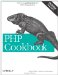# Recipe 2.7. Taking Logarithms

#### 2.7.1. Problem

You want to take the logarithm of a number.

#### 2.7.2. Solution

For logs using base e (natural log), use log( ):

`\$log = log(10);          // 2.30258092994`

For logs using base 10, use log10( ):

`\$log10 = log10(10);      // 1`

For logs using other bases, pass the base as the second argument to log( ):

`\$log2  = log(10, 2); // 3.3219280948874`

#### 2.7.3. Discussion

Both log( ) and log10( ) are defined only for numbers that are greater than zero. If you pass in a number equal to or less than zero, they return NAN, which stands for "not a number."

Documentation on log( ) at http://www.php.net/log and log10( ) at http://www.php.net/log10.PHP Cookbook: Solutions and Examples for PHP Programmers
ISBN: 0596101015
EAN: 2147483647
Year: 2006
Pages: 445

Similar book on Amazon